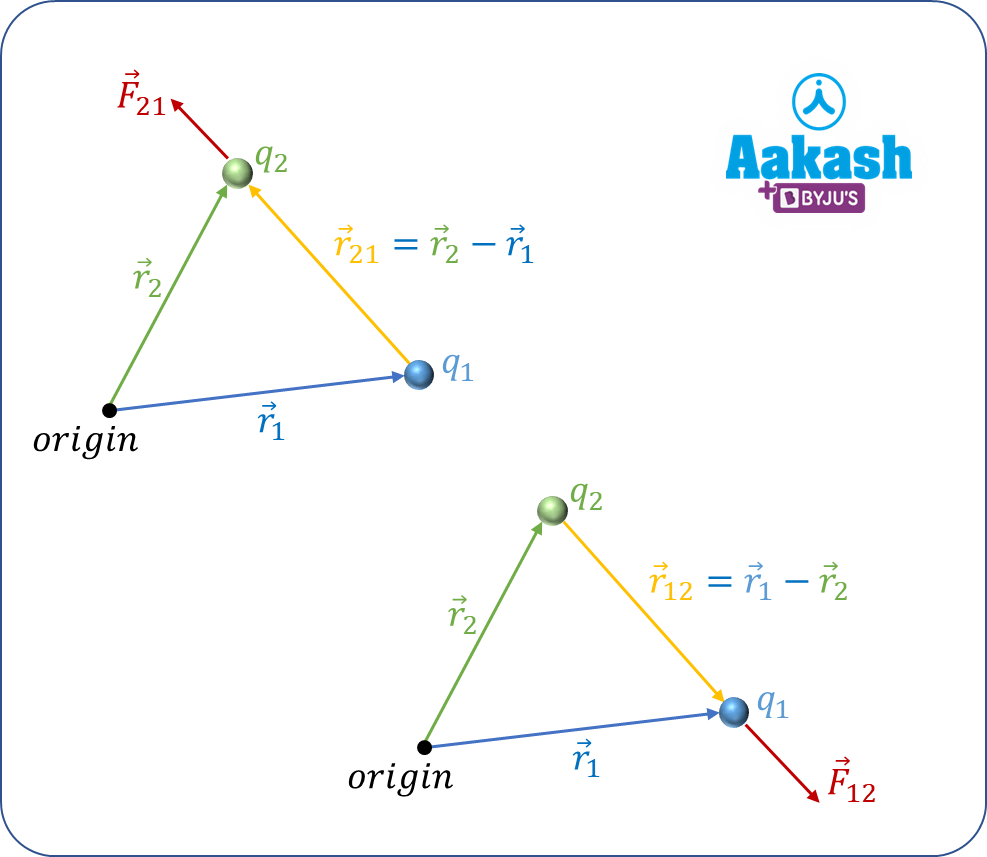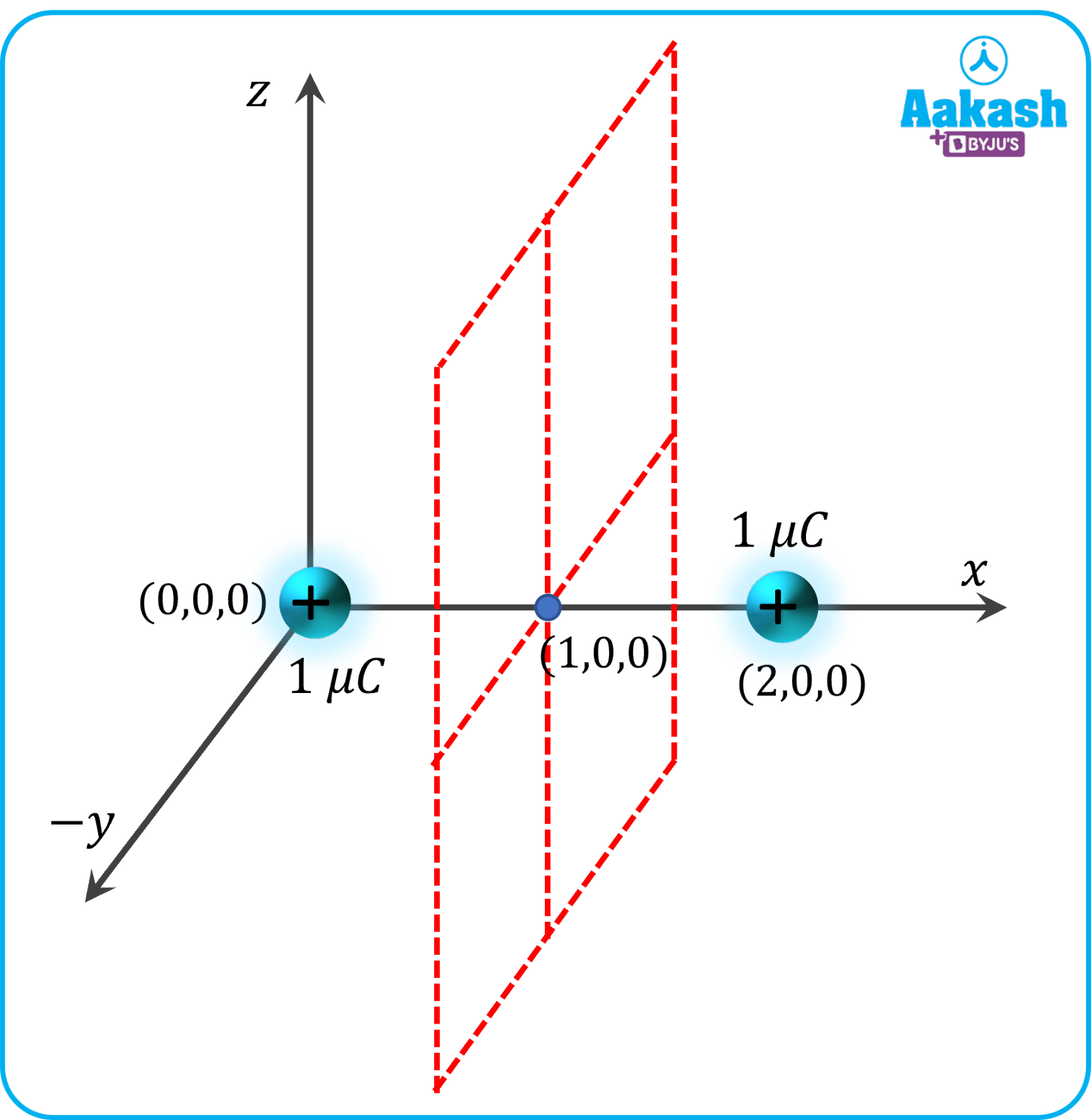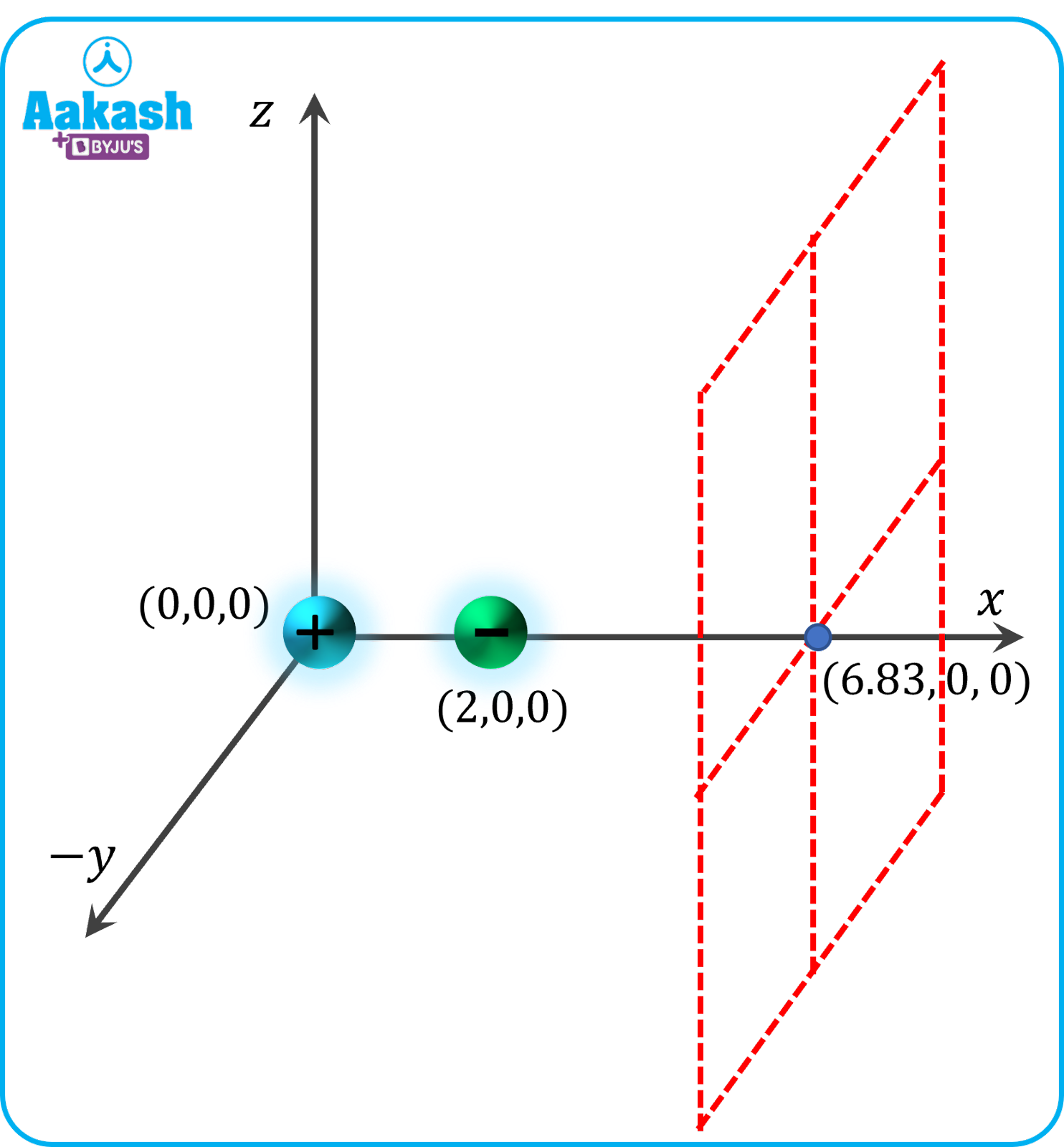• Call Now

1800-102-2727•

# Electrostatics - Electric charge, Conservation of charges, Coulomb’s Law, Electric Field, Practice Problems, FAQs

The branch of physics which studies stationary electric charges is electrostatics. In electrostatics no moving charges exist and a static equilibrium has been established. Moreover, as this phenomenon arises from the forces exerted by the electric charges on each other, it is defined by Coulomb's Law. Hence, to understand the concept of electrostatics, there is a need to be aware of electric charge and Coulomb’s Law.

Have you ever noticed that in the dry winter season when we rub our body with a plastic chair, the body hairs stand when we come closer to the plastic chair. This happens because of the static electricity. The frictional charge transfer between our body and the plastic chair gives rise to static electricity of opposite polarity between the plastic and the hairs. When two bodies of opposite charge come closer, they attract each other. Thus the hairs stand.

Table of content

• Electric charge
• Conservation of charges
• Coulomb’s Law
• Electric Field
• Practice Problems
• FAQs

## Electric charge

Electric charge or electrostatic charge is a basic property of subatomic particles. It is the main cause of electrostatic forces.

S.I Unit of Charge is Coulomb, C.

### Positively Charged Particles

These are the charged particles where the charge of the collective positive ions is more than that of the negative ions. In order to make the object/particle neutral, electrons from the surroundings (maybe some other object or even the ground) have to come to this object/particle.

### Negatively Charged Particles

These are the charged particles where the charge of the collective positive ions is less than that of the negative ions or electrons. In order to make the object/particle neutral, electrons or negative ions have to go out from this object/particle to the surroundings (maybe some other object or even the ground).

### Neutral Particles

Neutral particles have positively charged ions and negative ions or electrons in equal numbers. Every object has protons, neutrons and electrons. In case of a neutral object the number of positive ions equal the number of negative ions or free electrons.

## Conservation of charges

Conservation of Charge says, the total electric charge in an isolated system is always conserved. The net quantity of electric charge i.e., the amount of positive charge plus the amount of negative charge in an isolated system or in the universe as a whole, is always conserved.

The net charge of an object depends on the number of excess electrons or protons. These particles are the constituent of the total charges in a system. For a neutral body there is always the same number of electrons and protons in it.

Every stable atom is electrically neutral. It means it contains the same number of electrons outside the nucleus and the protons inside the nucleus. Atoms or the bodies can only have elementary charge in the multiples of whole numbers.

So, the smallest charge that a body or an atom can have is the charge of one electron or one proton. The charge a proton has is +1.6×10-19 C and for the electron the charge is –1.6× 10-19 C. So, the charge contained will be an integral multiple of ± 1.6×10-19 C.

## Coulomb’s Law

### Coulomb’s First Law

Bodies or particles having like charges repel each other and the bodies or particles with unlike charges, attract each other.

### Coulomb’s Second Law

The electrostatic force, whether it is force of attraction or of repulsion between two charged particles is directly proportional to the product of their charges and inversely proportional to the square of the distance between them.Let there are two point-charges having charges qand q2 at a distance ${\stackrel{\to }{r}}_{21}={\stackrel{\to }{r}}_{2}-{\stackrel{\to }{r}}_{1}$. The force acting on the charge q2 by the point charge qwill be ${\stackrel{\to }{F}}_{21}={\stackrel{\to }{F}}_{e}$

### Limitations of Coulomb's Law

Coulomb's law is to be applied only when the point charges are in a state of rest.

Coulomb's law is only applicable when the charged objects in consideration are point charges. In case of distributed charges of arbitrary shape, we can’t apply coulomb's law directly. In those cases, we have to take elementary pieces of the charged body and calculate.

## Electric Field ($\stackrel{\to }{E}$)

The electric field can be defined as the vector field of the electrical force by a unit charge exerted on a positive test charge at a state of rest at that point.

An electric field can be generated by an electric charge or by a time-varying magnetic field.

The electric field has the same direction and the magnitude of the electrostatic force which is due to a fixed charge or a set of fixed charges on a unit positive charge.

Let’s consider a test positive charge q0 at a distancefrom another charge q.

In that case the electric field atdue to the charge q is the force on the test charge q0 if q0 were a unit positive charge.

The force on q0 is
So, the electric field will be,
In case the charge q is a negative charge (i.e., -q) then
The unit of electric field in SI system is NC-1.

### Gauss’ Law:

The net flux through a closed surface is $\frac{1}{{\epsilon }_{0}}$ times the net charge enclosed by the surface.

From Gauss’s law, the net flux through a surface is given by,

${\varphi }_{net}=\frac{{q}_{enclosed}}{{\epsilon }_{0}}$

## Practice Problems

Q1. Let’s take a charged sphere made of copper of radius 1 m. The sphere is charged by 1 μC. What is the electric field at the centre of the sphere?

A. The charge of 1 μC will spread over the surface of the sphere. It will distribute over the surface and no charge will reside inside.

We can apply the Gauss law, $\int Eds=\frac{{q}_{in}}{ϵ}$

If we consider an imaginary sphere of radius r < 1 m,

So, the electric field at the centre is 0 NC-1

Q2. A copper sphere of radius 1 m is charged by 1 μC. What is the electric field at a distance 2 m from the centre?

A. The field outside the sphere can be calculated as the field due to a point charge 2 m away.

So, if we consider the field at 2 m as E then,

Q3. There are two point-charges of 1 μC are at (0m ,0m) and (2m,0m). Where on the x axis the electric field be zero?

A. The charges are of the same polarity. From the understanding of the geometry we can understand that the only possibility of the field becoming zero is when the point under consideration will lie in between the charges.

The electric field at a general point x,0 where 0 < x < 2, E can be given as,The above expression will become zero when x = 1 m

It means at the point (1m,0m) the field will be zero.

It is to be noted that, everywhere on the plane x = 1 m the electric field will be zero.

Q4. There are two point-charges of 2 C and -1μC are at (0m ,0m) and (2m,0m). Where on the x axis the electric field be zero?

A. The charges are of different polarity. From the understanding of the geometry we can understand that there is no possibility of the field becoming zero when the point under consideration will lie in between the charges.

The electric field at a general point x,0 x>2m E can be given as,The above expression is equal to zero when x- 8x + 8=0

Solving the equation, we get x=1.17m ,6.83m

As x>2m, x=6.83 m is only the possible solution.

So, at the point (6.83 m,0) the electric field will be zero.

It is to be noted that, everywhere on the plane x=6.83 m the electric field will be zero.

## FAQs

Q1. Let’s consider a soap bubble. The soap bubble was neutral. Now it is charged with +2 μC. Will its size remain the same?

A. The size of a soap bubble is a function of the excess pressure inside the bubble and the surface tension of the soap solution. When the soap bubble is given a charge of +2 μC, all the charge distributes itself evenly on the surface. Now because of the same nature of charge, every particle starts repelling each other. So, the size of the bubble increases.

Q2. A scientist claims that he is able to charge in a multiple of 1.6×10-21 C. Is he telling the truth?

A. Charging a body is all about creating an excess of electrons or deficient of electrons. Excess of electrons makes the body negatively charged. Deficiency of electrons makes a body positively charged. When there is neither deficiency nor excess of electrons, the body is neutral.

So, the magnitude of charge in a body can only be an integral multiple of the charge of electron i.e. 1.6×10-19 C. It is clear that the scientist is telling a lie as it is not possible to have a charge of 1.6×10-21 C. The value is 1100th of the charge in an electron which is not an integral multiple of the charge in an electron i.e., 1.6×10-19 C.

Q3. Let’s consider two copper spheres of the same radius. One of the spheres is solid and the other sphere is hollow. They are touched to a charged body and then separated. Which copper sphere is having a greater charge now?

A. We know copper is the conductor of electricity. So, any charge residing inside will come out and reside on the surface. This can be understood by the Gauss law. We can also understand this as follows. When the charges repel each other, the charges will try to position themselves as far as possible. Being a conductor, they are free to move and the best possible case is when the charges will be on the surface of the sphere. In both the cases, the charges will reside on the surface of the spheres. These copper spheres are charged from the same object. So, they will have an equal charge as all the charges reside on the surface of the material. Both the spheres have the same radius. They will have the same amount of charge as well.

Q4. Can a charge experience electrostatic force due to its own field?

A. The electric field is the electrical force by a charge exerted on a unit positive test charge at a particular point in the space when all the charges are in a state of rest.

If the charge is to have an electrical force due to its own field, there must be a force at the same position of the charge by the same charge itself.

The electric field due to a point charge q at a point at a distance r is .

If we are to derive the field at the same position, $\stackrel{\to }{r}$ → O i.e the field is not defined.

We can conclude that no charge will experience any force due to its own field.Talk to our expert
Resend OTP Timer =
By submitting up, I agree to receive all the Whatsapp communication on my registered number and Aakash terms and conditions and privacy policy